Reach Us+44 7456 035580
All submissions of the EM system will be redirected to Online Manuscript Submission System. Authors are requested to submit articles directly to Online Manuscript Submission System of respective journal.

# Performance Analysis of FSO Modulation Schemes with Diversity Reception Over Atmospheric Turbulence Channel

Wafaa MRS*

Al-Furat Al-Awsat Technical University, Babylon, Iraq

*Corresponding Author:
Wafaa MRS
Al-Furat Al-Awsat Technical University
Babylon, Iraq
E-mail: [email protected]

Received Date: 28/05/2016; Accepted Date: 08/08/2016; Published Date: 16/08/2016

Visit for more related articles at Research & Reviews: Journal of Engineering and Technology

## Abstract

Free space optical (FSO) communication is a cost effective, high bandwidth access systems. The fading of the transmitted signal that induced by the channel atmospheric turbulence is the major impairment in the FSO communication link which caused severe performance degradation for different optical modulation schemes. Spatial diversity is one of the atmospheric turbulence mitigation techniques that used for improving the FSO system performance. In this paper, the BER expressions of the PPM, PAM and OOK modulation schemes over FSO atmospheric turbulence channel with log-normal distribution are derived. A significant performance improvement of the system with a proposed receiver spatial diversity technique is observed with increasing the number of receiver photodetectors for various channel turbulence level. The performance comparison analysis of the PPM and PAM schemes is shown that for the same signal intensity power, PPM offered better BER performances, although PAM scheme is shown better bandwidth performances.

#### Keywords

Atmospheric turbulence, Free space optical, Log-normal distribution, Spatial diversity, Fading channel, Optical modulation schemes

#### Introduction

Although free space optical (FSO) communication has attracted a wide spectrum of applications since it is a license free and highly bandwidth access technique , it has to overcome many challenges that originate from free space propagation media. These challenges may include snow, fog, smog and aerosol scattering, which leave the terrestrial FSO communication link weak to adverse weather conditions. Atmosphere turbulence causing by atmospheric temperature and pressure inhomogeneity is the major impairment in FSO communication and this will lead to optical signal refractive index fluctuations . When the optical signal passes through such a turbulent atmosphere, it will experience random fluctuation in the intensity that’s lead to fluctuations in the received signals' amplitude and phase. This phenomenon is termed as scintillation . Degradation of the FSO communication performance is the direct effect of scintillation due to causing deep signal fades and thus increasing the bit error rate .

Many techniques are proposed to mitigate the effects of atmospheric turbulence including error control coding , adaptive optics , and diversity technique . Error control coding schemes may introduce a huge timing delay and efficiency performance degradation due to increasing the processing complexity and the number of redundant bits . Adaptive optics schemes are mainly used in astronomical applications. However, these schemes introduce lose in signal energy which can be compact through longer observation time. Thus, these methods are not suitable for real-time communication applications purposes . Receiver spatial diversity technique is based on using multiple separate photodetectors on the reception side, these photodetectors will simultaneously receive multiple copy of the transmitted signal which experience different channel fade effects, then combining these copies of the signal to mitigate the channel effects, and this will lead to increasing the signal to noise ratio .

The ultimate goal of any modulation scheme is to send as largest amount of data over the smallest spectral with least power needed. The Pulse Position Modulation (PPM), Pulse Amplitude Modulation (PAM) and On-Off Keying (OOK) are the most commonly used modulation techniques in FSO communication systems depending on the specific requirements of the certain optical system such as system simplicity, power and bandwidth efficiency .

In this paper, a proposed system based on receiver spatial diversity with selection combining (SelC) technique is introduced; the purpose of the proposed system is to mitigate the atmospheric turbulence channel effects on the optical signal that transmitted over the FSO channel. The BER expressions of the PPM, PAM and OOK modulation schemes over atmospheric turbulence channel with log-normal distribution are derived. The performance comparisons analysis for these three modulation schemes including the effect of different turbulence levels is presented. The performance comparisons include the effect of the number of receiver photodetectors, and the effect of variation of M-PPM, L-PAM modulation levels, on the system BER performance is presented. In addition, the performance comparison between the PPM and PAM schemes in term of the system bandwidth requirement is discussed in this work.

This paper is organized as follows: Section II includes an atmospheric turbulences description. Section III gives the system and channel models. In Section IV, the BER system performance expressions for the PPM, PAM and OOK modulation schemes with spatial diversity receiver are derived. Finally, results and conclusions are given in Section V and Section VI, respectively.

#### A Background Overview

The atmospheric turbulence phenomena degrade the performance of the FSO communications by causing a random variation of the received optical signal that leads to signal fluctuations. The atmospheric turbulence effects may include the electromagnetic scattering from the atmospheric molecules that causes optical energy redirection . The second effect is due to the density inhomogeneity in the atmosphere, caused by pressure and temperature fluctuations, which creates random variations of refraction index n throughout the optical signal propagation medium. These variations can be expressed as a sum of an average term and a fluctuating term as given in the following .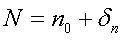(1)

where no is the average value of the refractive index and (δn) is the fluctuation component that is induced by the spatial variations of temperature and pressure in the atmosphere. The associated power spectral density Φn(k) of the refractive index fluctuations is described by the Kolmogorov model as the following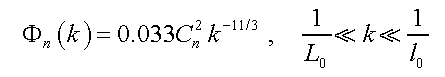(2)

where C2n is the refractive index structure parameter, k is the spatial wave number, lo is the inner scale of turbulence, and Lo is the outer scale of turbulence. The main effect of atmospheric turbulence is due to scintillation that causes random fluctuations in the amplitude and phase of the received optical signal. A common measure of scintillation is known as scintillation index (σ2sc ), that is given as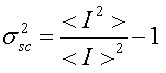(3)

where > I <is the received optical signal intensity. The Huffnagel-Valley Boundary (HVB) model is used for modeling the terrestrial FSO communication link, which can be described as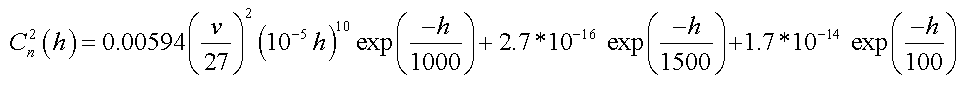(4)

Here h is the altitude in meters (m), and v is the wind velocity in meters per second (m/sec).

System and channel models

In this paper, a receiver spatial diversity with selection combining technique is proposed. Considering a single laser diode transmitter with A aperture area and N positive intrinsic negative (PIN) photodetectors at the receiver, the received power will be equal to received signal intensity. The aperture area of each detector in the N-photodetector receiver system is assumed to be (Ar/N), where Ar is the aperture area of the PIN photodetector under single transmitter single receiver (SISO) system. The SelC linear combiner compares all the received signals through its multiple branches and selects the branch with the highest signal intensity level, the output of the combiner is the signal with the highest intensity and this makes SelC technique less complexity compared to the maximum ratio combining (MRC) and equal gain combining (EGC) techniques. The additive white Gaussian noise (AWGN) models the noise channel. The received signal at the nth photodetector is given as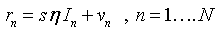(5)

where s is the transmitted information bits, η is the optical to electrical conversion efficiency of the receiver, vn is the additive white Gaussian noise with zero mean and variance of σ2v=N0/2. The fading channel coefffcient In which models the channel from the transmitter to the nth photodetector is given by(6)

where Io is the signal light intensity without turbulence and Xn is the identically distributed normal random variable with mean μx and variance σ2x. The probability density function (PDF) of the received signal over the log-normal channel model is given by(7)

where In is the received signal intensity in the presence of turbulence and I0 is the received signal intensity without the effect of turbulence, σ2x is the log-intensity variance and μx is the mean of log-intensity variance, which related to the scintillation index through the following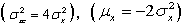. For spatial diversity system with N photodetectors, the total average received intensity power is given by.

Derivation of bar expressions for the receiver spatial diversity

Firstly, let’s consider the OOK modulation scheme at the transmitter and spatial diversity with N-PIN detector at the receiver, the probability of error in ideal channel condition i.e., in the absence of turbulence is given by .(8)

where Rp is the PIN detector responsivity and Rb is the system data rate. The noise variance σ2v is the summation of the shot noise and the thermal noise variances. The shot noise is caused by background light while the thermal noise is a result of thermally induced random fluctuations in the charge carriers in the resistive element of the photodetector . Q is the Gaussian-Q function, which is given by the following expression ,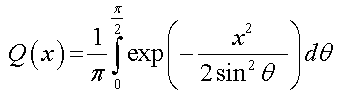(9)

The average BER can be obtained by averaging equation (8) over the PDF of In as: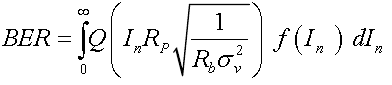(10)

The integration of equation (10) has no closed form solution. So, we have to solve it numerically based on Hermite polynomial which gives approximately the same value through the summation of its basis values which are roots and weights. The Hermite polynomial formula is given by .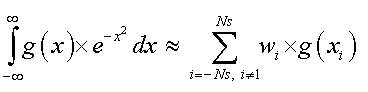(11)

whereand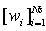are the roots and the weights of the Ns th order Hermite polynomial. It was shown in  that accurate results can be obtained if Ns ≥ 10. To apply the Hermite polynomial of equation (10), variable x is represented as the following :(12)

From equation (12) In and dIn in terms of x and dx from equation (12) as,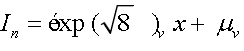(13)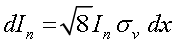(14)

By substituting equations (13) and (14) in equation (10), equation (10) can be rewritten as

(15)

Then,(16)

By appling the Hermite polynomial of equation (11) to equation (16), the BER of OOK modulation scheme over the FSO lognormal fading channel can be expressed as: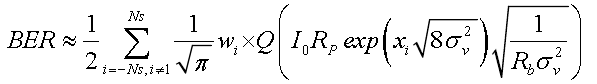(17)

Hence, BER expression of N-photodetector spatial diversity system over log-normal channel with OOK modulation scheme at the transmitter is given by:(18)

Secondly, to drive the BER of M-PPM scheme over FSO channel, we follow the same steps for driving the BER of the OOK modulation scheme starting with the BER of M-PPM scheme without turbulence which is given by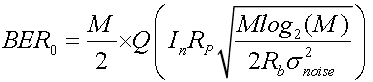(19)

where, M is the M-PPM modulation order, taking into account the FSO turbulence effects, the BER of M-PPM scheme is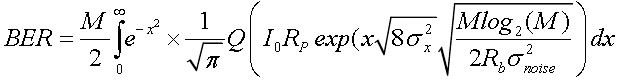(20)

Applying the Hermite polynomial of equation (11), the BER expression of M-PPM over the log-normal fading channel using PIN detector can be approximated as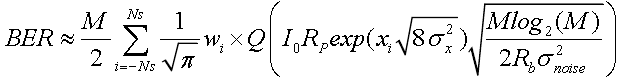(21)

Hence, the M-PPM final expression of bit error rate of N- PIN photodetector spatial diversity system is given by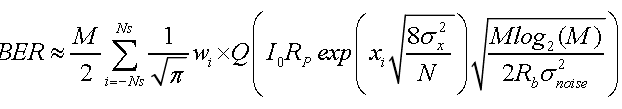(22)

Finley to find the BER expression of L-PAM scheme, we start with the L-PAM probability of error, without turbulence which is given by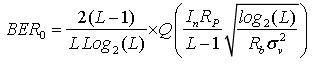(23)

where, L is the L-PPM modulation order, The BER of L-PAM over the FSO turbulence channel is given by,(24)

Applying the Hermite polynomial of equation (11), the BER expression of L-PAM over log-normal fading channel using PIN detector can be approximated as(25)

The final expression of the L-PAM bit error rate with N-photodetector spatial diversity system over log-normal FSO channel is given by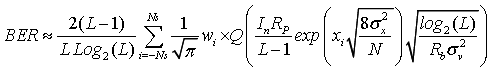(26)

Another important indicator to evaluate the performance of FSO communication systems over the turbulence channel is the system requirements bandwidth which measures how much data can be transmitted over the spectral frequency in terms of modulation order and data bit rate. The bandwidth of the M-ary PPM (BWM-PPM) scheme is given by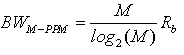While the bandwidth of L-ary PAM (BWL-PAM) scheme is given by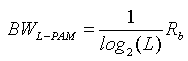(28)

The block diagram of the proposed system model is depicted in Figure 1, where the information bits are modulated using either OOK, M-PPM or L-PAM scheme. The laser diode is used to modulate the symbols. The transmitted light propagates over the log-normal fading channel. At the receiver, the PIN photodiode converts the light intensity into electrical current and Selection Combiner is used to estimate the transmitted information bits.

#### Results and Discussions

In the following analysis, it is assumed that the proposed system has N-PIN photodetectors with Rp=0.9 (A/W) responsivity, system data rate is (800 Mbps) and the order of Hermite polynomial is equation (20).

In Figure 2, the achieved BER performance of 2-PPM and 2-PAM schemes against the received light intensity (Io) at turbulence level (σ2x=0.3) and different number of photodetectors N is depicted. The results of Figure 2 indicate that the 2-PPM scheme achieved better BER performances than the PAM scheme for the different number of N. Where, for the case of 4-N detectors, PAM need extra (3.5 dB) to achieved (10-6) BER comparing with the PPM scheme for the same number of N. It is also clear that, the increasing of the number of receiver photodetector will lead to decreasing the BER for the same modulation scheme, where the 4-N PPM requires (1 dB), (1.5 dB), and (2 dB) less power than 3-N, 2-N, and 1-N PPM for (10-6) BER, while 4-N PAM requires (1 dB), (2 dB), and (3 dB) less power than 3-N, 2-N, and 1-N PAM for the same value of BER.

Figure 2: BER performance of 2-PPM, 2-PAM schemes against the received light intensity (Io) with (1,2,3,4-N).

The plotting of BER performance of OOK, M-PPM, L-PAM schemes against the received light intensity (Io) with 2-N photodetectors at trublunce level (σ2x=0.7) and various modulation orders M and L is shown in Figure 3. The results of Figure 3 clearly indi cate that the required (Io) to achieved particular BER is decreases with increasing the modulation order M in M-PPM scheme, while the required (Io) to obtain any desired BER is increases with the increasing the modulation order L in L-PAM scheme. In addition, it is also clear that the BER performance of OOK scheme is equal to the performance of 2-PPM. The 32-PPM requires (3 dB), (7 dB), (9.5 dB), and (13.5 dB) less power comparing with 16-PPM, 8-PPM, 4-PPM, and 2-PPM, for (10-6) BER, while 32-PAM requires (1.5 dB), (2.5 dB), (3 dB), and (3.5 dB) more power than 16-PAM, 8-PAM, 4-PAM, and 2-PAM for the (10-6) BER. The 32-PPM scheme requires (17.5 dB) less power than 32-PAM scheme to achieve BER of (10-6), which clearly indicates that the higher modulation order of the M-PPM scheme led to less BER performance comparing with other modulation schemes.

Figure 3: BER performance of OOK, M-PPM, L-PAM schemes against the received light intensity (Io) with (σ2x=0.7)

The required bandwidth performance comparison of the 2-PPM and 2-PAM schemes verses (Io) for 1-N,4-N and 8-N photodetector numbers is shown in Figure 4 with turbulence level (σ2x=0.9). It can be seen from the figure that with increasing the number of photodetectors N, the required (Io) will decrease to achieved bandwidth for both PPM and PAM schemes although the PAM scheme shows slightly better bandwidth performances than PPM for all value of N. The Figure 4 shows that 8-N PAM requires (15 dB) and (55 dB) less power than 4-N, 1-N PAM to achieve 1 GHz bandwidth, while the 8-N PPM requires (10 dB) and (50 dB) less power than 4-N, 1-N PPM to achieve the same bandwidth, these results indicate that PPM is less bandwidth efficiency than the of the PAM technique and the required power is increased with increasing the bandwidth value for the both modulation techniques.

Figure 4: Bandwidth performance of PPM, PAM schemes against the received light intensity (Io) with (σ2x=0.9).

#### Conclusions

This paper presents a performance analysis study for using receiver spatial diversity technique for the FSO modulation schemes OOK, M-PPM and L-PAM. The BER expressions of the three modulation techniques are derived under the log-normal distribution fading channel condition. A significant improvement in BER and bandwidth performances are achieved with increasing the number of receiver photodetectors. It was concluded that the M-PPM with higher modulation order has more efficient BER performance comparing with other modulation schemes of the same number of the photodetectors. It was also concluded that L-PAM scheme is more bandwidth efficiency comparing with M-PPM scheme for the same channel turbulence level.